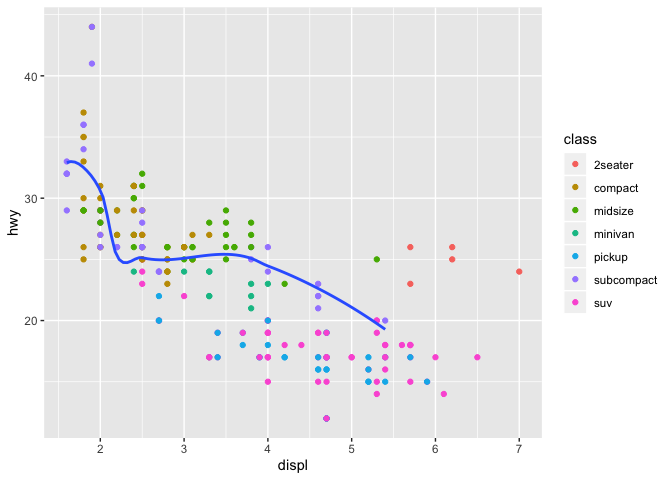# Grammar of Graphics with ggplot2 - Part 2

## Facets

• In some cases, the plot is clearer and more informative to be split into facets, subplots that each display one subset of the data.

• There are 2 main methods to use facets in ggplot2:
• facet_wrap()
• facet_grid()

### facet_wrap()

• Use facet_wrap() to facet your plot by a single variable.
• The first argument of facet_wrap: ~ followed by a variable name.
• The variable that you pass should be discrete (categorical).
library(tidyverse)
ggplot(data = mpg) +
geom_point(mapping = aes(x = displ, y = hwy)) +
facet_wrap(~ class, nrow = 2)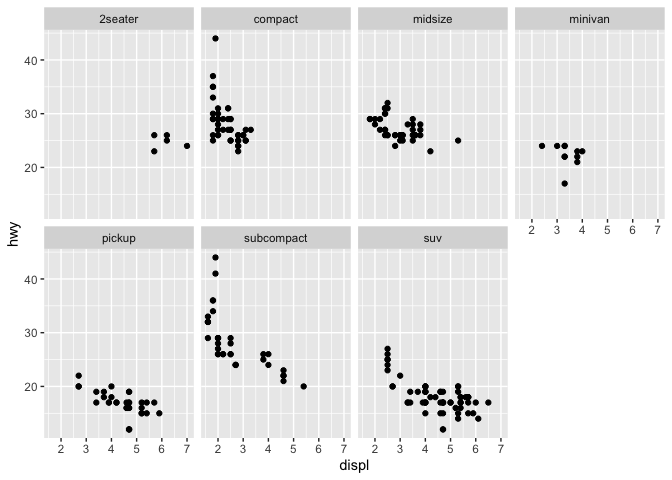### facet_grid()

• Use facet_grid() to facet your plot on the combination of two variables.
• The first argument is two variable names separated by a ~.
• Both of the variables should be discrete (categorical).
ggplot(data = mpg) +
geom_point(mapping = aes(x = displ, y = hwy)) +
facet_grid(drv ~ cyl)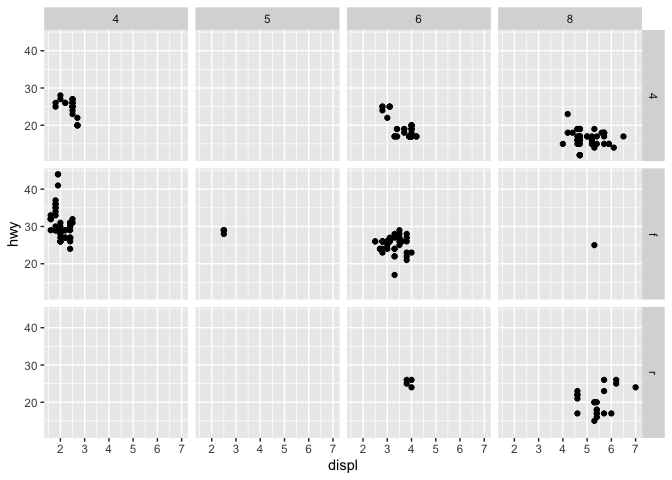• You can also replace one of the variable names with . to get a similar result as facet_wrap().

### Exercises

• Question: What happens if you facet on a continuous variable?

## Geometric Objects

• A geom is the geometrical object that a plot uses to represent data.
# scatterplot - point geom
p1.1 <- ggplot(data = mpg) +
geom_point(mapping = aes(x = displ, y = hwy))

# fitted smooth line - smooth geom
p1.2 <- ggplot(data = mpg) +
geom_smooth(mapping = aes(x = displ, y = hwy))

# use girdExtra to arrange multiple plots in a grid
library(gridExtra)
grid.arrange(p1.1, p1.2, nrow = 1)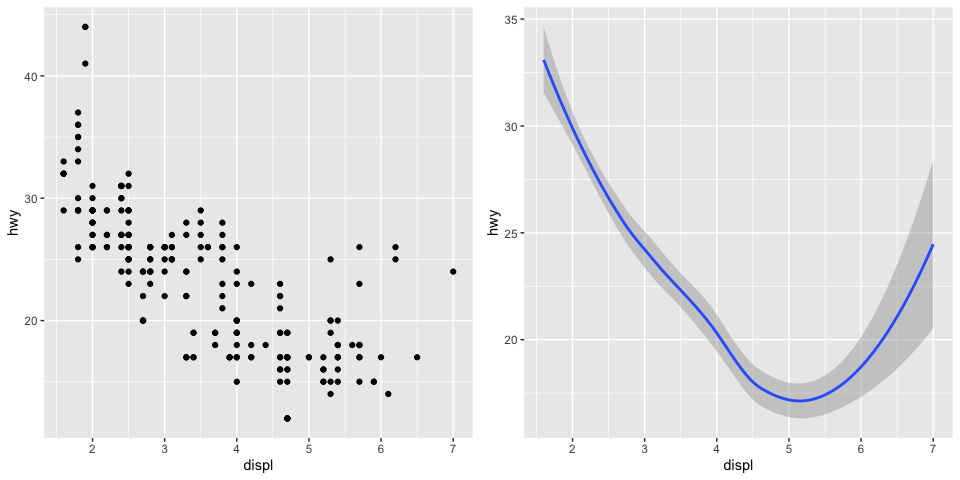• Both plots describe the same relationship, yet they use different geoms to plot.
• Use geom_...() function to specify the geom you want to use.

### geom_...() function

• Every geom function in ggplot2 takes a mapping argument.
• But not every aesthetic works with every geom.

• Example:
• shape is an aesthetic that does not work with geom_smooth()
• But geom_smooth() will draw a different line, with a different linetype, for each unique value of the variable that you map to linetype.
ggplot(data = mpg) +
geom_smooth(mapping = aes(x = displ, y = hwy, linetype = drv))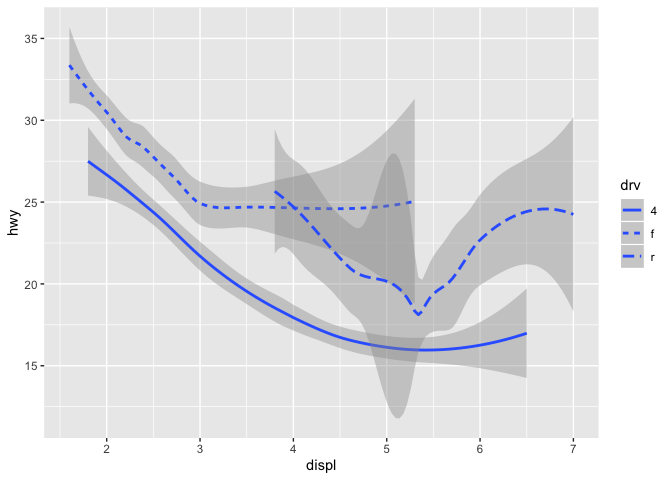### ggplot2 cheatsheet

• ggplot2 provides over 40 geoms!
• The best way to get a comprehensive view of the ggplot2 package is to take a look at the ggplot2 cheatsheet.
• The cheatsheet link is also available on the course website.

### group aesthetic

• Set the group aesthetic to a categorical variable to draw multiple objects (groups of rows shared the same value) in one plot.
p2.1 <- ggplot(data = mpg) +
geom_smooth(mapping = aes(x = displ, y = hwy))

p2.2 <- ggplot(data = mpg) +
geom_smooth(mapping = aes(x = displ, y = hwy, group = drv))

p2.3 <- ggplot(data = mpg) +
geom_smooth(
mapping = aes(x = displ, y = hwy, color = drv),
show.legend = FALSE)

grid.arrange(p2.1, p2.2, p2.3, nrow = 1)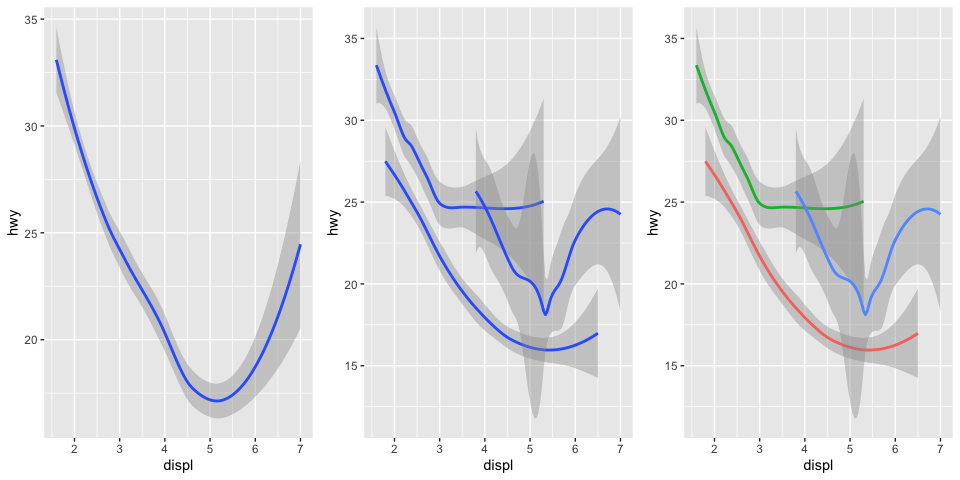### Multiple layers

• We can use both geoms at the same time!
ggplot(data = mpg, mapping = aes(x = displ, y = hwy)) +
geom_point(mapping = aes(color = drv)) +
geom_smooth(mapping = aes(linetype = drv, color = drv))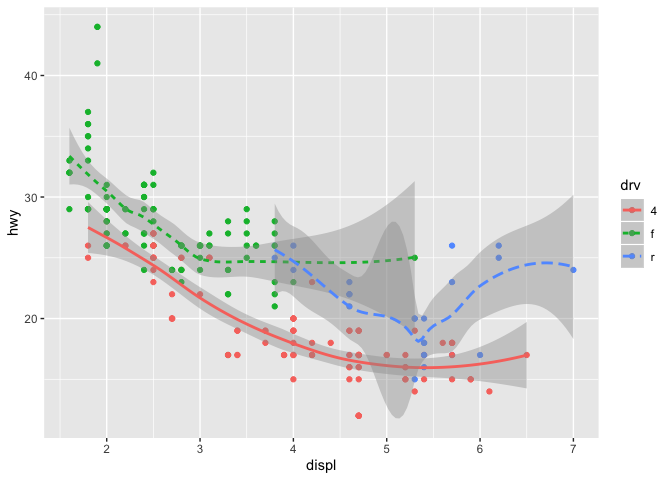• Note how I wrote the code above:
• How many layers are there?
• Where do I define the shared aesthetics of the two layers?
ggplot(data = mpg) +
geom_point(mapping = aes(x = displ, y = hwy)) +
geom_smooth(mapping = aes(x = displ, y = hwy))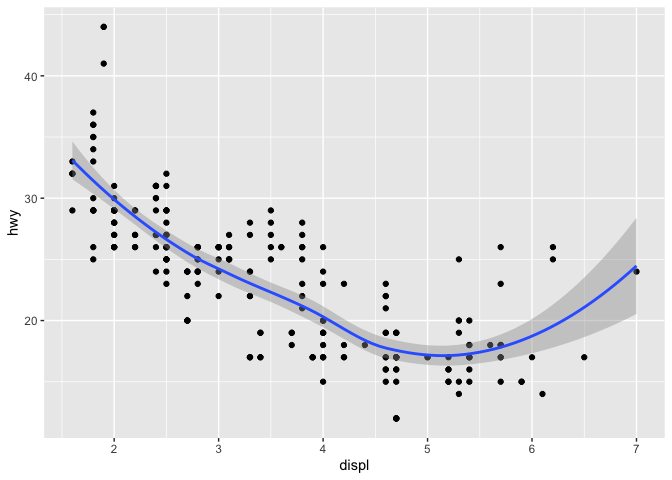• The above code can be written as:
ggplot(data = mpg, mapping = aes(x = displ, y = hwy)) +
geom_point() +
geom_smooth()• If you place mappings in a geom function, ggplot2 will treat them as local mappings for that layer only.
ggplot(data = mpg, mapping = aes(x = displ, y = hwy)) +
geom_point(mapping = aes(color = class)) +
geom_smooth()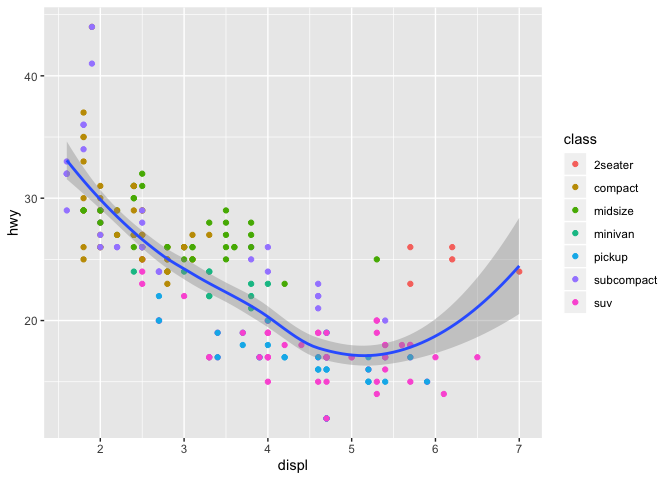• You can even specify different data for a layer!
ggplot(data = mpg, mapping = aes(x = displ, y = hwy)) +
geom_point(mapping = aes(color = class)) +
geom_smooth(data = filter(mpg, class == "subcompact"), se = FALSE)